# 15th LSI Design Contests・in Okinawa  Design Specification - 3-1

## 3-1. Discrete Fourier Transform

DFT (Discrete Fourier Transform) is the fourier transform on the discrete time domain, and this method is often used for the frequency analysis of a discrete digital signal in the digital signal processing. Now, we perform the frequency analysis of discrete time domain signal x[n] ( x[n] is complex values).

We obtain the N-sample signal in frequency domain (complex values) X, … , X[N-1] which use DFT to the N-sample signal in time domain x, … , x[N-1].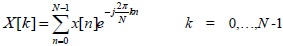(1.1)

Using the twiddle factor , the discrete Fourier coefficients are expressed as equation (1.2)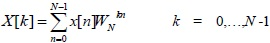(1.2)

The case of N=4 is shown using the matrix representation in equation (1.3).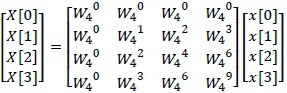(1.3)

Calculating equation (1.3) results are as following equations (1.4) 〜 (1.7).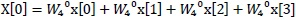(1.4)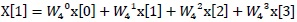(1.5)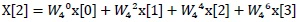(1.6)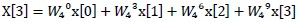(1.7)

According to equations (1.4) ~ (1.7), the N=4 DFT requires 16 times complex multipliers, and 12 times complex adders. Similarly, the N-point DFT requires N2 times complex multipliers, and N(N-1) times complex adders. The most of processing time which calculate the DFT using computer is the calculation time of the complex multipliers. If the number of complex multipliers is significant reduced, the processing speed of the DFT is expected to increase significantly.